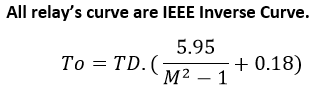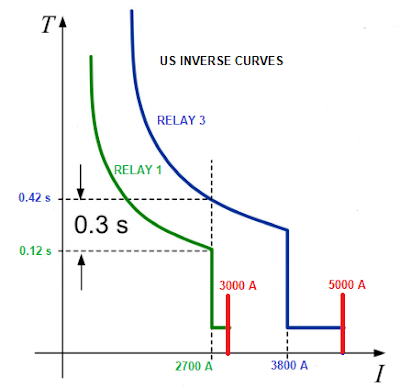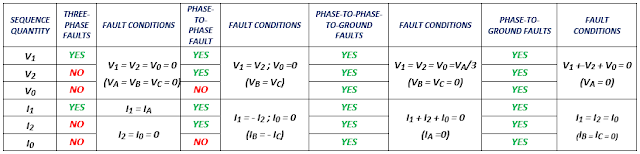## Saturday, January 30, 2021

### EXERCISE OF PHASE OVERCURRENT RELAY COORDINATION

Author: BSEE Hugo E Reyes

INTRODUCTION

The goal of this publication is to apply everything we learned in paper called "FUNDAMENTALS OF NON-DIRECTIONAL OVERCURRENT PROTECTION RELAYS":

OVERCURRENT RELAYS COORDINATION

The “IEEE STD 242 RECOMMENDED PRACTICE FOR PROTECTION AND COORDINATION OF INDUSTRIAL AND COMMERCIAL POWER SYSTEMS” section 15.6 outline seven steps that should be followed to make an overcurrent relays coordination.

In summary, these steps are:

a) Develop a detailed One-Line-Diagram (OLD).

b) Determine the various normal, temporary, and emergency operating configurations for the electrical power system.

c) Determine the load flow in the system.

d) Determine levels of short-circuits in each location:

-          Maximum and minimum momentary single and three-phase short-circuit currents (1/2 cycle network).

-          Maximum and minimum interrupting duty three-phase short-circuit currents (1.1/2 to 4 cycle network).

-          Maximum and minimum ground fault currents.

e) Determine the characteristics of protective devices and collects the Time-Current Characteristic (TCC) curves.

f) Collect the thermal damage limit curves from every electrical component, i.e. conductors, generators, transformers, motors, etc.

g) Determine the existing settings of the upstream and downstream overcurrent devices that have to be coordinated.

When every step has been done, we need to start the process at the loads, and select the branch circuit with the largest current setting, then establishing coordination relays pairs and work back to the power source.

EXERCISE 1 OF OVERCURRENT RELAYS COORDINATION

Consider a radial power system with two buses 1 and 2 where non-directional overcurrent relays are to be used (Figure 1).

-          The maximum short-circuit current available at Bus 2 is 5 kA,

-          The maximum load current at Bus 2 is 360 A

-          The minimum short-circuit current available at Bus 1 is 2 kA

RELAY 1 SETTINGS:

-         IPK-51P1P-1 = 4.6 A secondary, this is 276 A primary.

-         IPK-50P1P-1 = 45 A secondary, this is 2700 A primary.

-         Time Dial Multiplier equal to 0.5 (TD1 = 0.5).

Using the data given in Figure 1 we need to determine next Phase Relay 3 Settings:

-         IPK-50P1P-3 =?

-         IPK-51P1P-3 =?

-         TD3 =?SOLUTION

The purpose of the relay 1 is to provide primary protection to load 1, and the purpose of the relay 3 is to provide primary protection to the power line (266.8kcmil) and backup protection to the feeders that supplies load 1 and 2.

RELAY 3: RELEVANT DATA

-          Maximum Load = 360 A

-          Ampacity of the conductor 266.8 kcmil ACSR: 460 A @ 75ºC.

-          Maximum Short-circuit on the remote Bus 1: 3000 A (3kA).

-          The conductor damage current (I2. t) must be larger than the conductor ampacity at 75ºC.

a)      INSTANTANEOUS CURRENT PICKUP OF RELAY 3 (50P1P-3)

An instantaneous pickup current setting for backup protection is 125% the maximum current fault of the next downstream overcurrent relay 1.

IPK-50P1P-3 = 1.25 * IFMAX1-1 / CTR_3)

IPK-50P1P-3 = 1.25 * 3 000/100) 38 A

IPK-50P1P-3 = 38 A secondary (this is 3 800 A primary)

b)      CURRENT PICKUP OF RELAY 3 (51P1P-3)

The pickup current for the phase overcurrent relay 3 (51P1P-3) must to be set at a value greater than the maximum load current (IML) and lower than the minimum fault current in its circuit (IFMIN), so:

IML1 < IPK-51P1P-3 < IFMIN1

So,

360 A < IPK-51P1P-3 < 3 000 A

The Pickup Current Element (51-3) can be selected as:

IPK-51P1P-3 = 125% * IML = 1.25 * 360 A

IPK-51P1P-3 = 450 A (primary)

Here is better to select the conductor ampacity at 75ºC (460 A) as the pickup current setting than 450 A (1.25 times the maximum load (450 A).

Finally,

IPK-51P1P-3 = 460 A - primary

IPK-51P1P-3 = 4.6 A - secondary (value that the relay sees)

a)       TIME OPERATION  OF RELAY 3 (51P1T-3)

Since Relay 1 does not have to maintain selectively with any other relay, it can be made to operate the fastest.

In order to maintain selectively between Relay 1 and Relay 3, the following coordination criterion have to be met:

TO-R3  = TO-R1  + CTI

Where:

CTI: is the coordinating time interval

The typical value of the coordinating time interval (CTI) for Relay-Relay coordination is 0.30 seconds.

Now, knowing the Time Dial (TD1) of the Relay 1 that is equal to 0.5 seconds we can calculate the operating time of the Relay 1:

The Multiple of Pick-up Current of relay 1 (M1) would be:

M1 = IPK-50P1P-1 / IPK-51P1P-1

M1 = 45 / 4.6 = 9.8

Substituting these values (TD1 and M1), we get:

Then, the operating time of the relay 3 is:

TO-R3 = 0.12 s + 0.30 s = 0.42 seconds

Also, the Multiple of Pick-up Current of relay 3 (M3) would be:

M3 = IPK-50P1P-3 / IPK-51P1P-3

M1 = 38 / 4.6 = 8.3

Thus, we can now get the Time Dial of the relay 3 (TD3):

This finishes the settings of both relays.

In Figure 2 is shown the Time-Curve Characteristics (TCC) of Relays 1 and 3.Figure 2 TCCs of the Exercise 1.

SUMMARY OF RELAYS SETTINGS

RELAY 1:

-         IPK-51P1P-1 = 4.6 A secondary, this is 276 A primary.

-         IPK-50P1P-1 = 45 A secondary, this is 2 700 A primary.

-         TD1 = 0.5

-          TO-R1 = 0.12 s

RELAY 3:

-         IPK-51P1P-3 = 4.6 A secondary, this is 460 A primary.

-         IPK-50P1P-3 = 38 A secondary, this is 3 800 A primary.

-         TD1 = 1.55

-    TO-R3 = 0.42 s